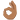# Complex numbers: Solve ##Z^2\bar{Z}=8i##

## Homework Statement:

Solve ##Z^2\bar{Z}=8i##

## Relevant Equations:

Complex numbers
Solve ##Z^2\bar{Z}=8i##

i am confused on how to proceed

i have tried to substitute ##z=a+ib## solve the conjugate and the square, then separate the real from the imaginary and put all in a system, but becomes too complicated

Related Precalculus Mathematics Homework Help News on Phys.org
fresh_42
Mentor
You could multiply it as ##(z\cdot \bar{z})\cdot z.## But you can also guess the solution.

##a^3+ia^2b+b^2a+ib^3=8i##
\begin{cases}
a^3+b^2a=0 \\
ia^2b+ib^3=8i
\end{cases}

fresh_42
Mentor
It is easier if you write ##a^3+b^2a=a\cdot (a^2+b^2)=0##, and ##a^2+b^2## cannot be zero.

It is easier if you write ##a^3+b^2a=a\cdot (a^2+b^2)=0##, and ##a^2+b^2## cannot be zero.
mmm ok so? sorry i'm not pickingfresh_42
Mentor
mmm ok so? sorry i'm not pickingYou have a product which multiplies to zero, so what does that mean for the factors?

no solution?

Another way is to expand ##(x+iy)^3## in a binomial expansion and compare real and imaginary parts in the equation.

no solution?
a and b are 0

Mark44
Mentor
a and b are 0
No
if you write ##a^3+b^2a=a\cdot (a^2+b^2)=0##, and ##a^2+b^2## cannot be zero.
You have a product which multiplies to zero, so what does that mean for the factors?
no solution?
No. To simplify what fresh_42 said, if ##a\cdot b = 0##, and b cannot be zero, what can you say about a?

at the moment nothing comes up on my mind sorry, completely blank

fresh_42
Mentor
at the moment nothing comes up on my mind sorry, completely blank
To get ##2\cdot x=0## you must have? And then, why is ##a^2+b^2 \neq 0##?

x must be 0
To get ##2\cdot x=0## you must have?
x is equal to 0
And then, why is ##a^2+b^2 \neq 0##?

fresh_42
Mentor
x must be 0

x is equal to 0

Yes, ##x=0##. So if ##a(a^2+b^2)=0## we must have either ##a=0## or ##a^2+b^2=0##. But squares of real numbers ##a,b## are never negative, so their sum isn't either. And there is only one way that ##a^2+b^2=0## can hold. Now does such a solution work for ##z^2\bar{z}=(a+ib)^2(a-ib)=\ldots = 8i ##?

DaveE
Gold Member
Have you learned the polar form Ae and how to multiply and conjugation with it? It's easier to see that way.

BTW, if this isn't familiar to you yet, then ignore it. You can solve it either way.

Yes, ##x=0##. So if ##a(a^2+b^2)=0## we must have either ##a=0## or ##a^2+b^2=0##. But squares of real numbers ##a,b## are never negative, so their sum isn't either. And there is only one way that ##a^2+b^2=0## can hold. Now does such a solution work for ##z^2\bar{z}=(a+ib)^2(a-ib)=\ldots = 8i ##?
so al so b is equal to 0

fresh_42
Mentor
so al so b is equal to 0
Yes, from ##a^2+b^2=0## we get ##a=b=0##. But then ##z=0## and this cannot be. Now that ##a^2+b^2\neq 0##, what do we get for ##a##?

Yes, from ##a^2+b^2=0## we get ##a=b=0##. But then ##z=0## and this cannot be. Now that ##a^2+b^2\neq 0##, what do we get for ##a##?i don't know, sorry

fresh_42
Mentor
We have ##a\cdot (a^2+b^2)=0## and ##a^2+b^2\neq 0.## That is the same as ##x\cdot 2= 0##. Just as if ##a^2+b^2## was ##2## and ##a## was ##x##. It isn't, but it is the same situation.

We have ##a\cdot (a^2+b^2)=0## and ##a^2+b^2\neq 0.## That is the same as ##x\cdot 2= 0##. Just as if ##a^2+b^2## was ##2## and ##a## was ##x##. It isn't, but it is the same situation.
a is 0

fresh_42
MentorYes. And now put ##a=0## into the second equation which we have:
\begin{cases}
ia^2b+ib^3=8i
\end{cases}Yes. And now put ##a=0## into the second equation which we have:
b= -2i?

fresh_42
Mentor
b= -2i?
No. You have written ##ib^3=8i## Now we divide both sides by ## i ## and have ##b^3 = 8.## No minus sign around. And ##b## is real! We set ##z=a+ib=0+ib=ib## and only need ##b##.

No. You have written ##ib^3=8i## Now we divide both sides by ## i ## and have ##b^3 = 8.## No minus sign around. And ##b## is real! We set ##z=a+ib=0+ib=ib## and only need ##b##.
yes sorry, was late for me and my brain very tired.##b=2## and ##Z=0+i2## therefore ##Z=2i##

•DaveE and fresh_42
mathwonk